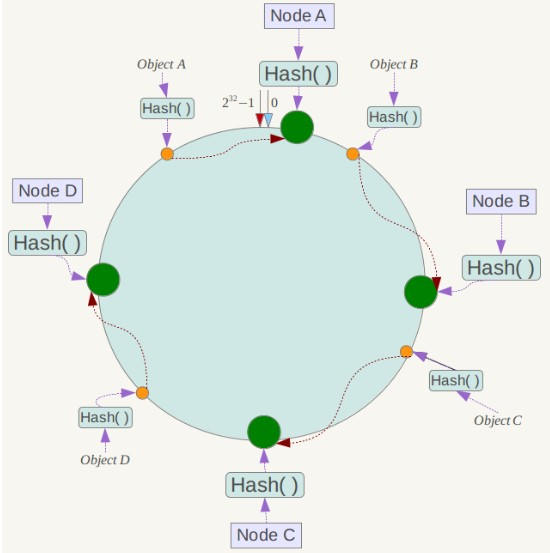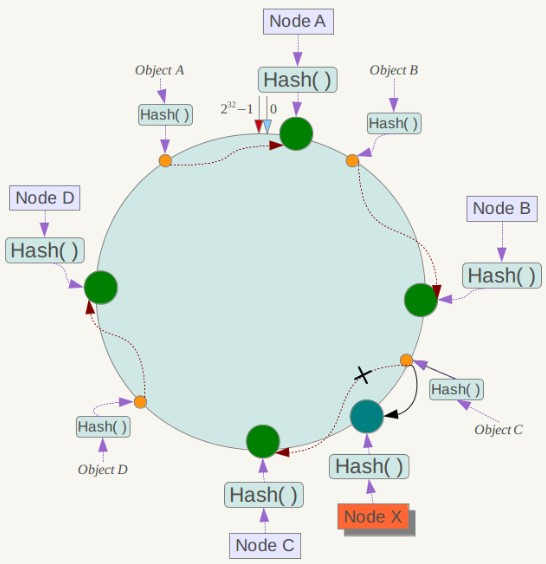# 一致性哈希算法

2019-01-29

## 1 概念“虚拟节点” 的hash计算可以采用对应节点的IP地址加数字后缀的方式。例如假设NODE1的IP地址为192.168.1.100。引入“虚拟节点”前，计算 cache A 的 hash 值：
Hash(“192.168.1.100”);

Hash(“192.168.1.100#1”); // NODE1-1
Hash(“192.168.1.100#2”); // NODE1-2

## 2 实现

/**
* 不带虚拟节点的一致性Hash算法
* @author
*
*/
public class ConsistentHashing {
// 待添加入Hash环的服务器列表
private static String[] servers = {"192.168.0.0:111", "192.168.0.1:111", "192.168.0.2:111","192.168.0.3:111", "192.168.0.4:111"};
// key表示服务器的hash值，value表示服务器的名称
// TreeMap默认是升序的，如果我们需要改变排序方式，则需要使用比较器：Comparator
private static SortedMap<Integer, String> sortedMap = new TreeMap<>();
// 程序初始化，将所有的服务器放入sortedMap中
static{
for(int i = 0; i < servers.length; i++) {
int hash = getHash(servers[i]);
System.out.println("[" + servers[i] + "]加入集合中, 其Hash值为" + hash);
sortedMap.put(hash, servers[i]);
}
System.out.println();
}

/**
* 得到应当路由到的结点
* @param data
* @return
*/
public static String getServer(String data) {
// 得到待缓存数据的Hash值
int hash = getHash(data);
// 得到大于该Hash值的所有Map
SortedMap<Integer, String> subMap = sortedMap.tailMap(hash);
// 第一个Key就是顺时针过去离node最近的那个结点
int i;
String res;
if (subMap.isEmpty()) {
i = sortedMap.firstKey();
res = sortedMap.get(i);
} else {
i = subMap.firstKey();
res = subMap.get(i);
}
return res;
}

/**
* 重写hash算法，为了让数据分布均匀
* @param data
* @return
*/
public static int getHash(String data) {
final int p = 16777619;
int hash = (int)2166136261L;
for (int i = 0; i < data.length(); i++){
hash = (hash ^ data.charAt(i)) * p;
}
hash += hash << 13;
hash ^= hash >> 7;
hash += hash << 3;
hash ^= hash >> 17;
hash += hash << 5;
// 如果算出来的值为负数则取其绝对值
if (hash < 0){
hash = Math.abs(hash);
}
return hash;
}

public static void main(String[] args) {
String[] datas = {"127.0.0.1:1111", "221.226.0.1:2222", "10.211.0.1:3333"};
for (int i = 0; i < datas.length; i++) {
System.out.println("[" + datas[i] + "]的hash值为" + getHash(datas[i]) + ", 被路由到结点[" + getServer(datas[i]) + "]");
}
}
}